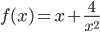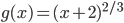### Day 36 - Extrema/Critical Numbers - 03.04.15

Update

Questions

Bell Ringer
1. Find the x-coordinate that has a horizontal tangent line for the following:1. -2

2. 0

3. 2

4. 3

5. none of the above

2. Does the point in #1 occur at a maximum or a minimum on the graph?

1. maximum

2. minimum

3. neither

3. Find the x-coordinate in which the following function does not have a derivative:1. 0

2. 2

3. -2

4. 1

5. none of the above

4. Does the point in #3 occur at a maximum or a minimum on the graph?

1. maximum

2. minimum

3. neither

Review
• N/A

Lesson

Exit Ticket
• N/A
Lesson Objective(s)
• How is the derivative used to locate the minimum and maximum values of a function on a closed interval?
Skills
1. Find critical numbers using differentiation.
2. Find extrema on a closed interval using differentiation.

#### In-Class Help Requests

Standard(s)
• APC.5
• Investigate derivatives presented in graphic, numerical, and analytic contexts and the relationship between continuity and differentiability.
• The derivative will be defined as the limit of the difference quotient and interpreted as an instantaneous rate of change.
• APC.6
• ​The student will investigate the derivative at a point on a curve.
• Includes:
• finding the slope of a curve at a point, including points at which the tangent is vertical and points at which there are no tangents
• using local linear approximation to find the slope of a tangent line to a curve at the point
• ​defining instantaneous rate of change as the limit of average rate of change
• approximating rate of change from graphs and tables of values.
• APC.7
• Analyze the derivative of a function as a function in itself.
• Includes:
• comparing corresponding characteristics of the graphs of f, f', and f''
• ​defining the relationship between the increasing and decreasing behavior of f and the sign of f'
• ​translating verbal descriptions into equations involving derivatives and vice versa
• defining the relationship between the concavity of f and the sign of f "
• APC.9
• Apply formulas to find derivatives.
• Includes:
• derivatives of algebraic and trigonometric functions
• derivations of sums, products, quotients, inverses, and composites (chain rule) of elementary functions
• derivatives of implicitly defined functions
• higher order derivatives of algebraic and trigonometric functions

Past Checkpoints
• N/A